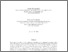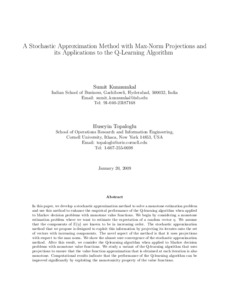A stochastic approximation method with max-norm projections and its applications to the Q-learning algorithm

Kunnumkal, S and Topaloglu, H (2010) A stochastic approximation method with max-norm projections and its applications to the Q-learning algorithm. ACM Transactions on Modeling and Computer Simulation, 20 (3).Preview Text (Postprint) sup_projection.pdf | Preview

Abstract

In this article, we develop a stochastic approximation method to solve a monotone estimation problem and use this method to enhance the empirical performance of the Q-learning algorithm when applied toMarkov decision problems with monotone value functions.We begin by considering a monotone estimation problem where we want to estimate the expectation of a random vector, nη. We assume that the components of Enη are known to be in increasing order. The stochastic approximation method that we propose is designed to exploit this information by projecting its iterates onto the set of vectors with increasing components. The novel aspect of the method is that it uses projections with respect to the max norm. We show the almost sure convergence of the stochastic approximation method. After this result, we consider the Q-learning algorithm when applied to Markov decision problems with monotone value functions. We study a variant of the Q-learning algorithm that uses projections to ensure that the value function approximation obtained at each iteration is also monotone. Computational results indicate that the performance of the Q-learning algorithm can be improved significantly by exploiting the monotonicity property of the value functions. © 2010 ACM.

ISB Creiators: ISB CreatorsORCiDKunnumkal, SUNSPECIFIED Article Max-norm projection; Q-learning; Stochastic approximation Applied Statistics and Computing LRC ISB 29 Oct 2014 04:50 14 Apr 2015 11:44 http://eprints.exchange.isb.edu/id/eprint/57 http://dx.doi.org/10.1145/1842713.1842715 http://www.sherpa.ac.uk/romeo/issn/1049-3301/View Item